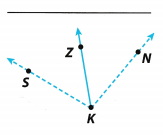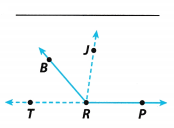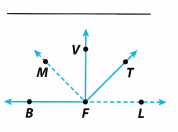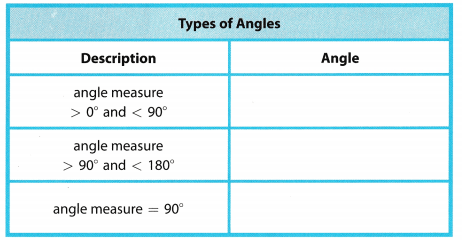# Texas Go Math Grade 6 Module 15 Answer Key Angles, Triangles, and Equations

Refer to our Texas Go Math Grade 6 Answer Key Pdf to score good marks in the exams. Test yourself by practicing the problems from Texas Go Math Grade 6 Module 15 Answer Key Angles, Triangles, and Equations.

## Texas Go Math Grade 6 Module 15 Answer Key Angles, Triangles, and Equations

Essential Question
How can you use angles, triangles, and equations to solve real-world problems?Complete these exercises to review skills you will need for this chapter.

Solve each equation using the inverse operation.

Question 1.
9p = 54 ________
Simplify the equation.
$$\frac{9 p}{9}$$ = $$\frac{54}{9}$$ divide both sides of the equation by 9
p = 6 value of the variable p

The value of the variable is 6.

Question 2.
m – 15 = 9 ________
Simplify the equation.
m – 15 + 15 = 0 + 15 add 15 to both sides of the equation
m = 24 value of the variable m

The value of the variable is 24.

Question 3.
$$\frac{b}{8}$$ = 4 __________
Simplify the eqsatton.
8 . $$\frac{b}{8}$$ = 4 . 8 multiply both sides of the equation by 8
b = 32 value of the variable b

The value of the variable is 32.

Question 4.
z + 17 = 23 _________
Simplify the equation.
z + 17 – 17 = 23 – 17 subtract 17 from both sides of the equation
z = 6 value of the variable z

The value of the variable is 6.

Give two names for the angle formed by the dashed rays.

Question 5.The angles formed by the rays on the diagram are ∠SKN and ∠K.

Angles are ∠SKN and ∠K.

Question 6.The angles formed by the rays on the diagram are ∠TRJ and ∠R.

Angles are ∠TRJ and ∠R.

Question 7.The angles formed by the rays on the diagram are ∠MFL and ∠F
Angles are ∠MFL and ∠F

Visualize Vocabulary
Use the ✓ words to complete the graphic. You will put one word in each oval.Understand Vocabulary

Complete the sentences using the review words.

Question 1.
A triangle that contains a right angle is a ________
A triangle that contains a right angle is a right triangle

The missing phrase is right triangle.

right triangle

Question 2.
An ______________________ has three congruent sides and three congruent angles.
An equilateral triangle has three congruent sides and three congruent angles.

The missing phrase is equilateral triangle.

equilateral triangle

Question 3.
The sides of triangles are ____. Where two lines meet to form an angle of a triangle is called a __________________